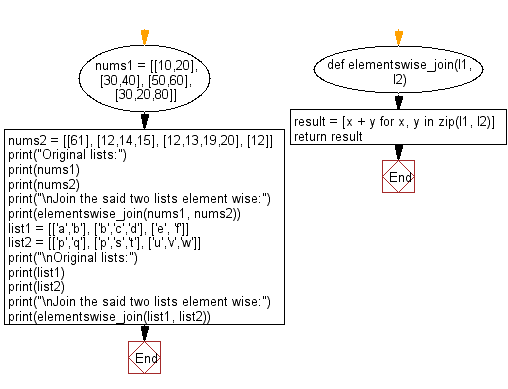﻿ Python: Join two given list of lists of same length, element wise - w3resource# Python: Join two given list of lists of same length, element wise

## Python List: Exercise - 154 with Solution

Write a Python program to join two given list of lists of same length, element wise.

Sample Solution:

Python Code:

``````def elementswise_join(l1, l2):
result = [x + y for x, y in zip(l1, l2)]
return result

nums1 = [[10,20], [30,40], [50,60], [30,20,80]]
nums2 = [, [12,14,15], [12,13,19,20], ]
print("Original lists:")
print(nums1)
print(nums2)
print("\nJoin the said two lists element wise:")
print(elementswise_join(nums1, nums2))

list1 = [['a','b'], ['b','c','d'], ['e', 'f']]
list2 = [['p','q'], ['p','s','t'], ['u','v','w']]
print("\nOriginal lists:")
print(list1)
print(list2)
print("\nJoin the said two lists element wise:")
print(elementswise_join(list1, list2))
```
```

Sample Output:

```Original lists:
[[10, 20], [30, 40], [50, 60], [30, 20, 80]]
[, [12, 14, 15], [12, 13, 19, 20], ]

Join the said two lists element wise:
[[10, 20, 61], [30, 40, 12, 14, 15], [50, 60, 12, 13, 19, 20], [30, 20, 80, 12]]

Original lists:
[['a', 'b'], ['b', 'c', 'd'], ['e', 'f']]
[['p', 'q'], ['p', 's', 't'], ['u', 'v', 'w']]

Join the said two lists element wise:
[['a', 'b', 'p', 'q'], ['b', 'c', 'd', 'p', 's', 't'], ['e', 'f', 'u', 'v', 'w']]
```

Flowchart:## Visualize Python code execution:

The following tool visualize what the computer is doing step-by-step as it executes the said program:

Python Code Editor:

Have another way to solve this solution? Contribute your code (and comments) through Disqus.

What is the difficulty level of this exercise?

Test your Python skills with w3resource's quiz

﻿

## Python: Tips of the Day

Floor Division:

When we speak of division we normally mean (/) float division operator, this will give a precise result in float format with decimals.

For a rounded integer result there is (//) floor division operator in Python. Floor division will only give integer results that are round numbers.

```print(1000 // 300)
print(1000 / 300)```

Output:

```3
3.3333333333333335```